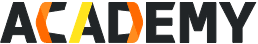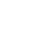#New User FREE Learning Path

Step 1: Claim the New User Benefit

Step 2: Redeem FREE Clouders to learn

Step 3: Get a FREE ACA Certification Exam

Operate and Manage a Cloud Server

Free

## Course Overview

#### In this course, we review Machine Learning and AI from top to bottom. Starting with an overview of the history of the field, we then move on to basic concepts and processes in machine learning. From there, we give detailed explanations of common algorithms in both general Machine Learning and other fields such as natural language processing (NLP). By the end of the course, students are not only familiar with common algorithms, but will have gained valuable hands-on experience with TensorFlow, a common open source AI and Machine Learning framework, as well as Machine Learning Platform for AI (PAI), Alibaba Cloud’s sophisticated but simple drag-and-drop Machine Learning tool.

In this course, we will introduce you to a wide variety of Machine Learning and AI material, including:

• The early history of machine learning and artificial intelligence
• Common machine learning algorithms and their applications
• Common deep learning tools and methods (Neural Networks, TensorFlow)
• Natural Language Processing: algorithms and applications
• Hands-on practice with TensorFlow and Alibaba Cloud’s Machine Learning Platform for AI (PAI)

## Target AudienceDevelopersSolution ArchitectsData ScientistsStudents

## Chapter 1: Machine Learning Overview: Concepts and History

 Course List Learning Objectives Video Course Course Content Lecture 1: Machine Learning and Artificial Intelligence Gain a simple understanding of Machine Learning and AI at a high level Lecture 2: Bacis Machine Learning Concepts and Processes Understand Machine Learning techniques at a high level Lecture 3: Classification of Machine Learning Methods Understand the concept, essence and mainstream technology of cloud native Lecture 4: Development history of Machine Learning Understand the early history of Machine Learning and AI techniques Quiz Test for the whole chapter and get your certification

## Chapter 2: Introduction to Machine Learning Algorithms

 Course List Learning Objectives Video Course Course Content Lecture 1: Perceptron Understand the perceptron algorithm Lecture 2: KNN (K-nearest Neighbors) Understand the KNN algorithm Lecture 3: Naïve Bayes Understand the Naïve Bayes algorithm Lecture 4: Decision Tree Understand the Decision Tree algorithm Lecture 5: Hierarchical Clustering Understand the Hierarchichal Clustering algorithm Lecture 6: K-means Clustering Understand the K-Means Clustering algorithm Lecture 7: Hands-on (lab) section Try out some basic Machine Learning algorithms hands-on Quiz Test for the whole chapter and get your certification

## Chapter 3: Neural Network Basics and Deep Learning

 Course List Learning Objectives Video Course Course Content Lecture 1: Neural Networks: Basic Concepts Understand Neural Networks at a high level Lecture 2: Neural Networks: Structure Understand the architecture of Neural Networks at a high level Lecture 3: Neural Networks: Optimization Understand optimization algorithms that are used with Neural Networks Lecture 4: Deep Learning: Basic Concepts Understand the general ideas behind so-called "Deep Learning" Lecture5: Convolutional Neural Networks and their Applications Understand the applications of CNNs, one of the most common types of Neural Network Lecture 6: Recurrent Neural Networks and their Applications Understand the applications of RNNs Lecture 7: Hands-on practice Gain hands-on practice with Neural Networks Quiz Test for the whole chapter and get your certification

## Chapter 4: Natural Language Processing

 Course List Learning Objectives Video Course Course Content Lecture 1: Statistical Language Model Understand statistical language models Lecture 2: Word2Vec Understand the Word2Vec model Lecture 3: Transformer Understand the Transformer model Lecture 4: BERT Understand the BERT language model Lecture 5: Sentiment Analysis Understand the basics of sentiment analysis Lecture 6: Chatbots Understand the basics of intelligent chatbots Lecture 7: Machine Translation Understand the basics of Machine Translation Quiz Test for the whole chapter and get your certification

## Chapter 5: Introduction to TensorFlow

 Course List Learning Objectives Video Course Course Content Lecture 1: Architecture and Working Principles Understand how TensorFlow works at a high level Lecture 2: Basic Syntax Understand TensorFlow's grammar and syntax Lecture 3: Development Process Understand how to set up TensorFlow and begin writing code Lecture 4: Development history of Machine Learning Understand the basics of Neural Network model development Quiz Test for the whole chapter and get your certification

## Chapter 6: Alibaba Cloud Machine Learning Platform for AI (PAI)

 Course List Learning Objectives Video Course Course Content Lecture 1: Introduction to PAI Understand what PAI is and what it can do Lecture 2: Visual (Studio) Development With PAI Understand how to use PAI's visual development interface Lecture 3: Notebook Development With PAI Understand how to use PAI's Jupyter notebook interface Lecture 4: Application: Image Classification See how PAI can be used to classify images Lecture 5: Application: Sentiment Analysis See how PAI can be used to build a sentiment analysis tool Lecture 6: Application: Product Recommendations See how PAI can be used to build recommender systems Quiz Test for the whole chapter and get your certification